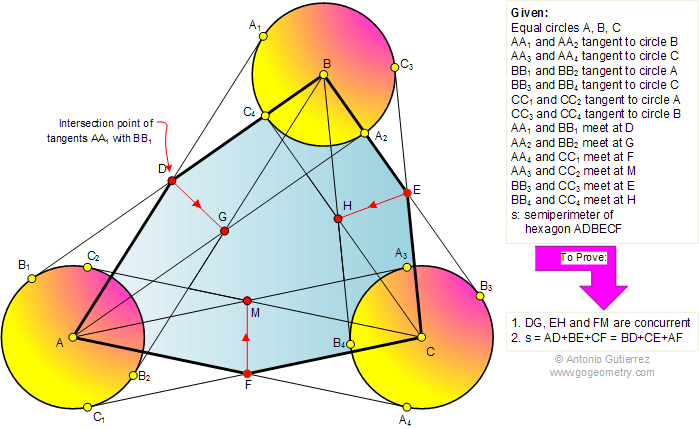Problem 345. Equal circles, Tangents, Concurrent lines, Hexagon, Semiperimeter. Level: High School, College, SAT Prep.

The figure shows equal circles A, B, and C. AA1, AA2, CC3, and CC4 are tangents to circle B, AA3, AA4, BB3, and BB4 are tangents to circle C, and BB1, BB2, CC1, and CC2 are tangents to circle A. Points D, E, F, G, H, and M are the intersection points of the tangents AA1 with BB1, BB3 with CC3, AA4 with CC1, AA2 with BB2, BB4 with CC4, and AA3 with CC2, respectively. Prove that (1) The extension of DG, EH, and FM are concurrent, (2) If s is the semiperimeter of the hexagon ADBECF, then s = AD + BE + CF = BD + CE + AF.Geometry problem solving is one of the most challenging skills for students to learn. When a problem requires auxiliary construction, the difficulty of the problem increases drastically, perhaps because deciding which construction to make is an ill-structured problem. By “construction,” we mean adding geometric figures (points, lines, planes) to a problem figure that wasn’t mentioned as "given."